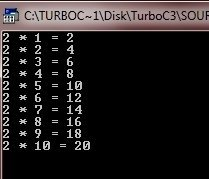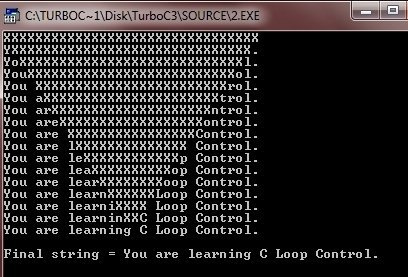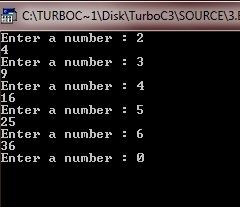# C for Loop

Here is the general form of the for loop in C language.

```for(initialization; condition; increment)
{
statements;
}```

Here initialization occurs first but only once, then checks the condition, if the conditions is true, then the loop control statements will executed, otherwise not. And then, the loop variable will be incremented or decremented, then checks the condition again, if the condition is true, then the loop control statements is again executed, otherwise not. This action is continued until the condition becomes false. Now concentrate on the following example program.

## C for Loop Example

Here are some example programs given that illustrates the C for loop.

```/* C for Loop example
* This program illustrates the concepts
* of C for loop
*/

#include<stdio.h>
#include<conio.h>
void main()
{
int num = 2;
int i;
clrscr();

for(i=1; i<=10; i++)
{
printf("%d * %d = %d", num, i, num*i);
printf("\n");
}

getch();
}```

Here is the sample output of this C program which prints the table of 2:Here is one more example demonstrating for loop in C programming.

```/* C for Loop Example Program
* This program illustrates multiple loop
* control variables
*/

#include<stdio.h>
#include<conio.h>
#include<string.h>

void conv(char *targ, char *src);

void main()
{
char target = "XXXXXXXXXXXXXXXXXXXXXXXXXXXXXXXX";
clrscr();

conv(target, "You are learning C Loop Control.");
printf("\nFinal string = %s\n", target);

getch();
}
/* this function copies one string into another string.
* It copies characters to both the ends,
* converging at the middle
*/
void conv(char *targ, char *src)
{
int i, j;
printf("%s\n", targ);
for(i=0, j=strlen(src)-1; i<=j; i++, j--)
{
targ[i] = src[i];
targ[j] = src[j];
printf("%s\n", targ);
}
}```

Here is the sample run of this C program:Here is another example program also illustrates for loop in C language.

```/* C for loop example program
* To quit this program, just enter 0
*/

#include<stdio.h>
#include<conio.h>

int sqrnum(int);
void prompt(void);

void main()
{
int t;
clrscr();

{
sqrnum(t);
}

getch();
}
void prompt(void)
{
printf("Enter a number : ");  // to quit, enter 0
return 0;
}
{
int num;
scanf("%d", &num);
return num;
}
int sqrnum(int num)
{
printf("%d\n", num*num);
return num*num;
}```

Here is the sample output of this C program, press 0 to quit the program.### More Examples

Here are some more examples that you can go for:

Tools
Calculator